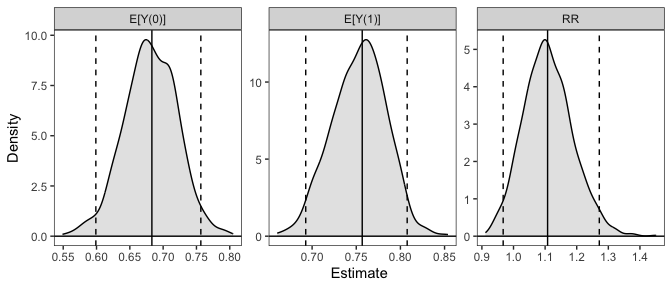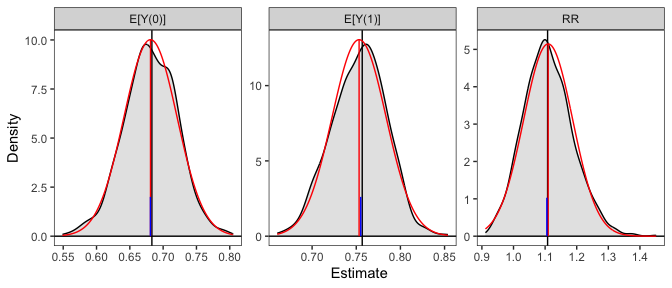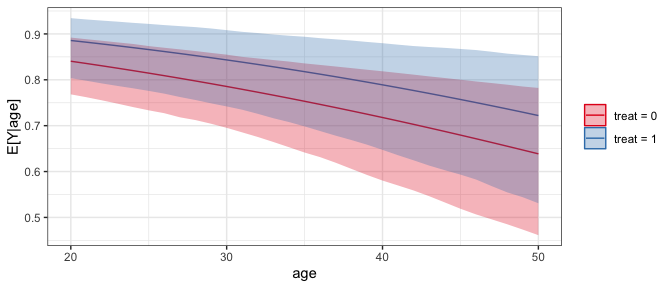# `clarify`: Simulation-Based Inference for Regression Models

`clarify` implements simulation-based inference for computing functions of model parameters, such as average marginal effects and predictions at representative values of the predictors. See the `clarify` website for documentation and other examples. `clarify` was designed to replicate and expand on functionality previously provided by the `Zelig` package.

## Installation

`clarify` can be installed from CRAN using

``install.packages("clarify")``

You can install the development version of `clarify` from GitHub with

``````install.packages("remotes")
remotes::install_github("iqss/clarify")``````

## Example

Below is an example of performing g-computation for the average treatment effect on the treated (ATT) after logistic regression to compute the average causal risk ratio and its confidence interval. First we load the data (in this case the `lalonde` dataset from `MatchIt`) and fit a logistic regression using functions outside of `clarify`:

``````library(clarify)

data("lalonde", package = "MatchIt")

# Fit the model
fit <- glm(I(re78 == 0) ~ treat * (age + educ + race + married +
nodegree + re74 + re75),
data = lalonde, family = binomial)``````

Next, to estimate the ATT risk ratio, we simulate coefficients from their implied distribution and compute the effects of interest in each simulation, yielding a distribution of estimates that was can summarize and use for inference:

``````# Simulate coefficients from a multivariate normal distribution
set.seed(123)
sim_coefs <- sim(fit)

# Marginal risk ratio ATT, simulation-based
sim_est <- sim_ame(sim_coefs, var = "treat", subset = treat == 1,
contrast = "RR", verbose = FALSE)

sim_est
#> A `clarify_est` object (from `sim_ame()`)
#>  - Average marginal effect of `treat`
#>  - 1000 simulated values
#>  - 3 quantities estimated:
#>  E[Y(0)] 0.2944381
#>  E[Y(1)] 0.2432432
#>  RR      0.8261270

# View the estimates, confidence intervals, and p-values
summary(sim_est, null = c(`RR` = 1))
#>         Estimate 2.5 % 97.5 % P-value
#> E[Y(0)]    0.294 0.220  0.384       .
#> E[Y(1)]    0.243 0.199  0.360       .
#> RR         0.826 0.619  1.391    0.79

# Plot the resulting sampling distributions
plot(sim_est)``````Below, we provide information on the framework `clarify` uses and some other examples. For a complete vignette, see `vignette("clarify")`.

## Introduction

Simulation-based inference is an alternative to the delta method and bootstrapping for performing inference on quantities that are functions of model parameters. The delta method involves multiple assumptions: 1) the model coefficients are normally distributed, 2) the resulting quantity of interest is normally distributed, and 3) the first-order approximation to the variance of the desired estimator is equal to the true variance. When these assumptions are incorrect, which is especially likely when the quantity of interest is a complicated nonlinear function of the model coefficients, the resulting inferences can be inaccurate. Bootstrapping is one solution to this problem that does not require any of the above assumptions for valid nonparametric inference (though other assumptions are required); however, it is computationally intensive because the original model needs to be fit many times, and any problems with the model that are only apparent in some bootstrap samples (e.g., failure to converge, perfect prediction) can make using bootstrapping challenging.

Simulation-based inference provides a compromise to these two methods: it is more accurate than the delta method because it does not require assumptions 2) and 3) (though it still relies on the central limit theorem to assume the coefficients are normally distributed), and it is faster and more stable than bootstrapping because the model only needs to be fit once. Simulation-based inference involves simulating model coefficients from their multivariate distribution using their estimated values and covariance from a single model fit to the original data, computing the quantities of interest from each set of model coefficients, and then performing inference using the resulting distribution of the estimates as their sampling distribution. Confidence intervals can be computed using the percentiles of the resulting sampling distribution, and p-values can be computed by inverting the confidence intervals. Alternatively, if the resulting sampling distribution is normally distributed, its standard error can be estimated as the standard deviation of the estimates and normal-theory Wald confidence intervals and p-values can be computed. The methodology of simulation-based inference is explained in King, Tomz, and Wittenberg (2000).

`clarify` was designed to provide a simple, general interface for simulation-based inference and includes a few convenience functions to perform common tasks like computing average marginal effects. The primary functions of `clarify` are `sim()`, `sim_apply()`, `summary()`, and `plot()`. These work together to create a simple workflow for simulation-based inference.

• `sim()` simulates model parameters from a fitted model
• `sim_apply()` applies an estimator to the simulated coefficients, or to the original object but with the new coefficients inserted
• `summary()` produces confidence intervals and p-values for the resulting estimates
• `plot()` produces plots of the simulated sampling distribution of the resulting estimates

There are also some wrappers for `sim_apply()` for performing some common operations: `sim_ame()` computes the average marginal effect of a variable, mirroring `marginaleffects::comparisons()` and `marginaleffects::marginaleffects()`; `sim_setx()` computes predictions at typical values of the covariates and differences between them, mirroring `Zelig::setx()` and `Zelig::setx1()`; and `sim_adrf()` computes average dose-response functions. `clarify` also offers support for models fit to multiply imputed data with the `misim()` function.

In the example above, we used `sim_ame()` to compute the ATT, but we could have also done so manually using `sim_apply()`, as demonstrated below:

``````# Write a function that computes the g-computation estimate for the ATT
ATT_fun <- function(fit) {
d <- subset(lalonde, treat == 1)
d\$treat <- 1
p1 <- mean(predict(fit, newdata = d, type = "response"))
d\$treat <- 0
p0 <- mean(predict(fit, newdata = d, type = "response"))
c(`E[Y(0)]` = p0, `E[Y(1)]` = p1, `RR` = p1 / p0)
}

# Apply that function to the simulated coefficient
sim_est <- sim_apply(sim_coefs, ATT_fun, verbose = FALSE)

sim_est
#> A `clarify_est` object (from `sim_apply()`)
#>  - 1000 simulated values
#>  - 3 quantities estimated:
#>  E[Y(0)] 0.2944381
#>  E[Y(1)] 0.2432432
#>  RR      0.8261270

# View the estimates, confidence intervals, and p-values;
# they are the same as when using sim_ame() above
summary(sim_est, null = c(`RR` = 1))
#>         Estimate 2.5 % 97.5 % P-value
#> E[Y(0)]    0.294 0.220  0.384       .
#> E[Y(1)]    0.243 0.199  0.360       .
#> RR         0.826 0.619  1.391    0.79

# Plot the resulting sampling distributions
plot(sim_est, reference = TRUE, ci = FALSE)``````The plot of the simulated sampling distribution indicates that the sampling distribution for the risk ratio is not normally distributed around the estimate, indicating that the delta method may be a poor approximation and the asymmetric confidence intervals produced using the simulation may be more valid. Note that the estimates are those computed from the original model coefficients; the distribution is used only for computing confidence intervals, in line with recommendations by Rainey (2023).

If we want to compute the risk difference, we can do that using `transform()` on the already-produced output:

``````#Transform estimates into new quantities of interest
sim_est <- transform(sim_est, `RD` = `E[Y(1)]` - `E[Y(0)]`)
summary(sim_est, null = c(`RR` = 1, `RD` = 0))
#>         Estimate   2.5 %  97.5 % P-value
#> E[Y(0)]   0.2944  0.2199  0.3841       .
#> E[Y(1)]   0.2432  0.1994  0.3602       .
#> RR        0.8261  0.6192  1.3908    0.79
#> RD       -0.0512 -0.1379  0.0927    0.79``````

We can also use `clarify` to compute predictions and first differences at set and typical values of the predictors, mimicking the functionality of `Zelig`’s `setx()` and `setx1()` functions, using `sim_setx()`:

``````# Predictions across age and treat at typical values
# of the other predictors
sim_est <- sim_setx(sim_coefs, x = list(age = 20:50, treat = 0:1),
verbose = FALSE)

#Plot of predicted values across age for each value of treat
plot(sim_est)``````See `vignette("Zelig", package = "clarify")` for more examples of translating a `Zelig`-based workflow into one that uses `clarify` to estimate the same quantities of interest.

`clarify` offers parallel processing for all estimation functions to speed up computation. Functionality is also available for the analysis of models fit to multiply imputed data. See `vignette("clarify")` for more details.

King, G., Tomz, M., & Wittenberg, J. (2000). Making the Most of Statistical Analyses: Improving Interpretation and Presentation. American Journal of Political Science, 44(2), 347–361. https://doi.org/10.2307/2669316

Rainey, C. (2023). A careful consideration of CLARIFY: Simulation-induced bias in point estimates of quantities of interest. Political Science Research and Methods, 1–10. https://doi.org/10.1017/psrm.2023.8